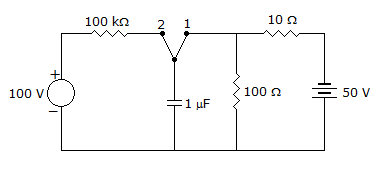# Electronics and Communication Engineering - Exam Questions Papers

31.

Which of the following counters can be used to divide the clock frequency of a microprocessor by 5?

 A. 3 bit counter B. 5 bit counter C. mod 3 counter D. mod 5 counter

Explanation:

No answer description available for this question. Let us discuss.

32.

In the given circuit, the switch has been in position 1 for quite a long time. At t = 0 the switch is moved to position 2. At this position what is the time constant?A. 0.1 s B. 1 s C. 0.11 s D. 1.11 s

Explanation:

No answer description available for this question. Let us discuss.

33.

A divide by 78 counters can be realized by using

 A. six mod-13 counters B. thirteen mod-6 counters C. one mod 13 counter followed by one mod-6 counter D. thirteen mod-13 counters

Explanation:

No answer description available for this question. Let us discuss.

34.

The process of extension of a single-crystal surface by growing a film in such a way that the added atoms form a continuation of the single-crystal structure is called

 A. Ion implantation B. Chemical vapour deposition C. Electroplating D. Epitaxy

Explanation:

No answer description available for this question. Let us discuss.

35.

Which of the following are the non-linear application of op-amp?

1. Current-to-voltage converter
2. Comparator
3. Peak detector
4. Limiter
Select the correct answer from the codes given below:

 A. 1, 2 and 3 B. 2, 3 and 4 C. 1, 3 and 4 D. 1, 2 and 4